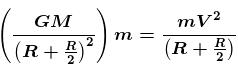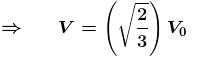Courses

# Central Forces NAT Level – 1

## 10 Questions MCQ Test Topic wise Tests for IIT JAM Physics | Central Forces NAT Level – 1

Description
This mock test of Central Forces NAT Level – 1 for IIT JAM helps you for every IIT JAM entrance exam. This contains 10 Multiple Choice Questions for IIT JAM Central Forces NAT Level – 1 (mcq) to study with solutions a complete question bank. The solved questions answers in this Central Forces NAT Level – 1 quiz give you a good mix of easy questions and tough questions. IIT JAM students definitely take this Central Forces NAT Level – 1 exercise for a better result in the exam. You can find other Central Forces NAT Level – 1 extra questions, long questions & short questions for IIT JAM on EduRev as well by searching above.
*Answer can only contain numeric values
QUESTION: 1

### The change in the value of g at a height h above the surface of the earth is the same as at a depth d below that surface of earth. When both d and h are much smaller than the radius of earth, then, d = αh. Find the value of  α.

Solution:

The gravitational field inside the earth is given by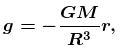where r is the distance from centre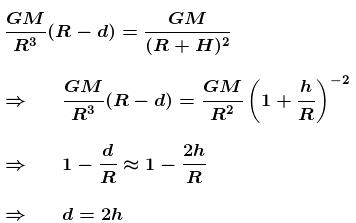*Answer can only contain numeric values
QUESTION: 2

### Energy required to move a body of mass m  from an orbit of radius 2R  to 3R  is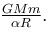Find the value of  α

Solution: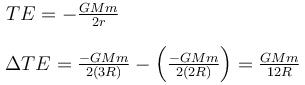*Answer can only contain numeric values
QUESTION: 3

### A satellite in a circular orbit of radius r has time period of  4hrs. A satellite with orbital radius of 4r around the same planet will have a time period of in hours.

Solution:

Radius of orbit of the first satellite

R1 = R

Time period of the first satellite T = 4 hrs. and radius of orbit of second satellite = 4r
The time period of satellite is given by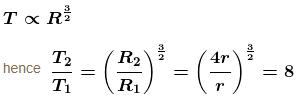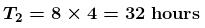*Answer can only contain numeric values
QUESTION: 4

The minimum and maximum distances of a satellite from the centre of earth are 2R and 4R respectively, where R is the radius of earth and M is the mass of the earth. The radius of curvature at the point of minimum distance is λR.  Find the value of λ.

Solution:

Applying conservation of angular momentum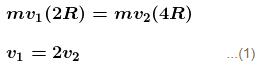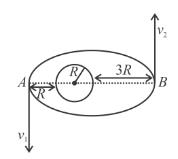From conservation of energy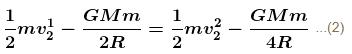Solving Eqs. (1) and (2), we get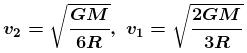If r is the radius of curvature at point A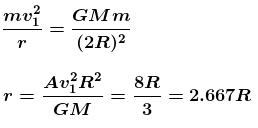*Answer can only contain numeric values
QUESTION: 5

Maximum height reached by a rocket fired with a speed equal to 50% of the escape velocity from Earth’s surface is R/α. Find the value of α.

Solution: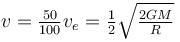Applying energy conservation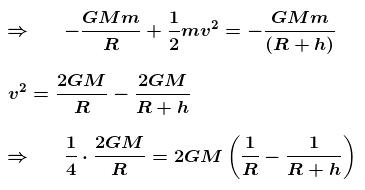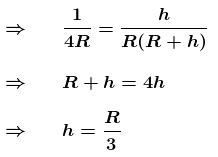*Answer can only contain numeric values
QUESTION: 6

Two satellites S1 and S2 of equal masses revolves in the same sense around a heavy planet in coplanar circular orbit of radii R and 4R. Find the value of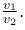Solution:

V=√(GM/r)
⇒ V1/V= (4/1)1/2
⇒ V1/V2 = 2/1

*Answer can only contain numeric values
QUESTION: 7

Radius of the earth is R and the means density is ρ. Find out the gravitational potential at the earth’s surface απGρR2.  Find the value of  α.

Solution: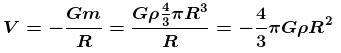*Answer can only contain numeric values
QUESTION: 8

A body which is initially at rest at a height R above the surface of the earth of radius R, falls freely towards the earth. Find out its velocity (in m/s) on reaching the surface of earth.

Take = 10m/s2 and = 6400km.

Solution:

Potential energy at ground surface
Potential energy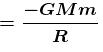potential energy at a height of R is
potential energy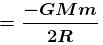When a body comes to ground
Loss in potential energy = Gain in kinetic energy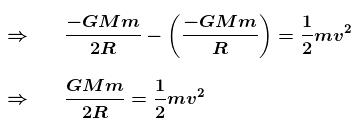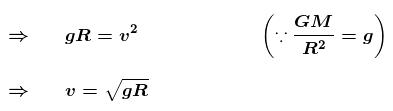*Answer can only contain numeric values
QUESTION: 9

A particle is projected from the surface of earth with an initial speed of 4.0km/s. Find the maximum height (in kms) attained by the particle.

Solution:

Radius of earth = 6400km and  the maximum height attained by the particle is,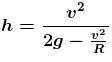and   g = 9.8 m/s2
Substituting the value,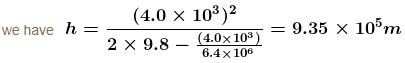or h = 935km

*Answer can only contain numeric values
QUESTION: 10

The orbital velocity of an artificial satellite in a circular orbit just above the earth’s surface is V0. The value of orbital velocity for another satellite orbiting at an altitude of half of earth’s radius is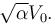Find the value of  α.

Solution:

For first satellite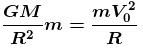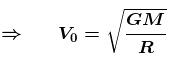For second satellite